International
Tables for
Crystallography
Volume B
Reciprocal space
Edited by U. Shmueli

International Tables for Crystallography (2006). Vol. B. ch. 2.5, pp. 281-282   | 1 | 2 |

## Section 2.5.2.5. Kinematical diffraction formulae

J. M. Cowleya

#### 2.5.2.5. Kinematical diffraction formulae

| top | pdf |

• (1) Comparison with X-ray diffraction. The relations of real-space and reciprocal-space functions are analogous to those for X-ray diffraction [see equations (2.5.2.2), (2.5.2.10)and (2.5.2.15)]. For diffraction by crystals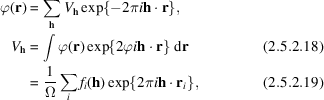where the integral of (2.5.2.18)and the summation of (2.5.2.19)are taken over one unit cell of volume (see Dawson et al., 1974).

Important differences from the X-ray case arise because

 (a) the wavelength is relatively small so that the Ewald-sphere curvature is small in the reciprocal-space region of appreciable scattering amplitude; (b) the dimensions of the single-crystal regions giving appreciable scattering amplitudes are small so that the shape transform' regions of scattering power around the reciprocal-lattice points are relatively large; (c) the spread of wavelengths is small (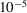or less, with no white-radiation background) and the degree of collimation is better (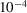to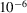) than for conventional X-ray sources. As a consequence of these factors, single-crystal diffraction patterns may show many simultaneous reflections, representing almost-planar sections of reciprocal space, and may show fine structure or intensity variations reflecting the crystal dimensions and shape.

• (2) Kinematical diffraction-pattern intensities are calculated in a manner analogous to that for X-rays except that

• (a) no polarization factor is included because of the small-angle scattering conditions;

• (b) integration over regions of scattering power around reciprocal-lattice points cannot be assumed unless appropriate experimental conditions are ensured.

For a thin, flat, lamellar crystal of thickness H, the observed intensity is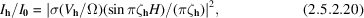where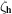is the excitation error for the h reflection and Ω is the unit-cell volume.

For a single-crystal diffraction pattern obtained by rotating a crystal or from a uniformly bent crystal or for a mosaic crystal with a uniform distribution of orientations, the intensity is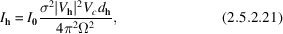where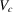is the crystal volume and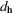is the lattice-plane spacing. For a polycrystalline sample of randomly oriented small crystals, the intensity per unit length of the diffraction ring is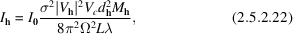where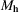is the multiplicity factor for the h reflection and L is the camera length, or the distance from the specimen to the detector plane. The special cases of oblique texture' patterns from powder patterns having preferred orientations are treated in IT C (2004, Section 4.3.5).

• (3) Two-beam dynamical diffraction formulae: complex potentials including absorption. In the two-beam dynamical diffraction approximation, the intensities of the directly transmitted and diffracted beams for transmission through a crystal of thickness H, in the absence of absorption, are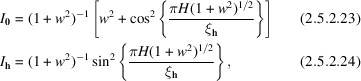where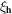is the extinction distance,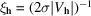, and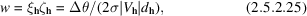where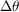is the deviation from the Bragg angle.

For the case that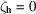, with the incident beam at the Bragg angle, this reduces to the simple Pendellösung expression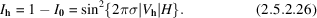The effects on the elastic Bragg scattering amplitudes of the inelastic or diffuse scattering may be introduced by adding an out-of-phase component to the structure amplitudes, so that for a centrosymmetric crystal,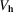becomes complex by addition of an imaginary component. Alternatively, an absorption function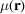, having Fourier coefficients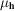, may be postulated so that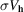is replaced by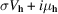. Theare known as phenomenological absorption coefficients and their validity in many-beam diffraction has been demonstrated by, for example, Rez (1978).

The magnitudesdepend on the nature of the experiment and the extent to which the various inelastically or diffusely scattered electrons are included in the measurements being made. If measurements are made of purely elastic scattering intensities for Bragg reflections or of image intensity variations due to the interaction of the sharp Bragg reflections only, the main contributions to the absorption coefficients are as follows (Radi, 1970):

 (a) from plasmon and single-electron excitations,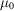is of the order of 0.1 V0 and, for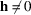, is negligibly small; (b) from thermal diffuse scattering;is of the order of 0.1 Vh and decreasing more slowly thanwith scattering angle. Including absorption effects in (2.5.2.26)for the casegives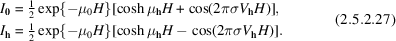The Borrmann effect is not very pronounced for electrons because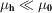, but can be important for the imaging of defects in thick crystals (Hirsch et al., 1965; Hashimoto et al., 1961). Attempts to obtain analytical solutions for the dynamical diffraction equations for more than two beams have met with few successes. There are some situations of high symmetry, with incident beams in exact zone-axis orientations, for which the many-beam solution can closely approach equivalent two- or three-beam behaviour (Fukuhara, 1966). Explicit solutions for the three-beam case, which displays some aspects of many-beam character, have been obtained (Gjønnes & Høier, 1971; Hurley & Moodie, 1980).

### ReferencesInternational Tables for Crystallography (2004). Vol. C. Mathematical, physical and chemical tables, edited E. Prince, 3rd ed. Dordrecht: Kluwer Academic Publishers.Google ScholarDawson, B., Goodman, P., Johnson, A. W. S., Lynch, D. F. & Moodie, A. F. (1974). Some definitions and units in electron diffraction. Acta Cryst. A30, 297–298.Google ScholarFukuhara, A. (1966). Many-ray approximations in the dynamical theory of electron diffraction. J. Phys. Soc. Jpn, 21, 2645–2662.Google ScholarGjønnes, J. & Høier, R. (1971). The application of non-systematic many-beam dynamic effects to structure-factor determination. Acta Cryst. A27, 313–316.Google ScholarHashimoto, H., Mannami, M. & Naiki, T. (1961). Dynamical theory of electron diffraction for the electron microscope image of crystal lattices. I. Image of single crystals. II. Image of superposed crystals (moiré pattern). Philos. Trans. R. Soc. London, 253, 459–516.Google ScholarHirsch, P. B., Howie, A., Nicholson, R. B., Pashley, D. W. & Whelan, M. J. (1965). Electron microscopy of thin crystals. London: Butterworths.Google ScholarHurley, A. C. & Moodie, A. F. (1980). The inversion of three-beam intensities for scalar scattering by a general centrosymmetric crystal. Acta Cryst. A36, 737–738.Google ScholarRadi, G. (1970). Complex lattice potentials in electron diffraction calculated for a number of crystals. Acta Cryst. A26, 41–56.Google ScholarRez, P. (1978). In Electron diffraction 1927–1977, edited by P. J. Dobson, J. B. Pendry & C. J. Humphreys, pp. 61–67. Inst. Phys. Conf. Ser. No. 41. Bristol, London: Institute of Physics.Google Scholar